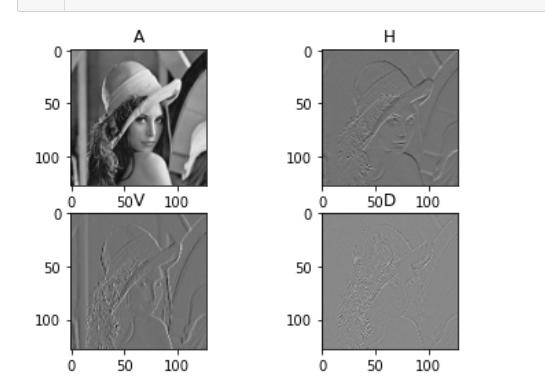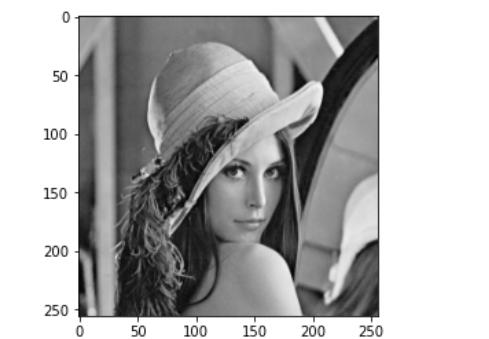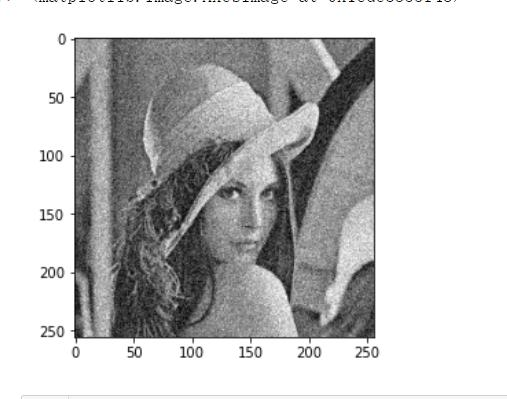• 多小波分解重构处理图像
千次阅读
2021-05-13 15:19:08

MATLAB实现图像的分解和重构命令有两种：

## 第一种是一层小波分解dwt2

[CA,CH,CV,CD]=dwt2(X,'wname');

其中，dwt2表示离散小波变换；X为输入参数，是图像；'wname'是小波名字；输出变量CA为低低频分解信息，刻画原始图像的逼近信息；CH为低高频分解信息，刻画原始图像的横向细节；CV为高低频分解信息，刻画原始图像的垂直细节；CD为高高频分解信息，刻画原始图像的对角线上的细节。

[CA,CH,CV,CD]=dwt2(X,Lo_D,Hi_D);

输入参数还可以是低通滤波器和高通滤波器

## 对应的重构命令为：

X=idwt2(CA,CH,CV,CD,'wname');

X=idwt2(CA,CH,CV,CD,Lo_D,Hi_D);

X=idwt2(CA,CH,CV,CD,Lo_D,Hi_D，'mode',MODE);

## 第二种是多层小波分解wavedec2

[C,S]=wavedec2(X,N,'wname');

其中，wavedec2为多层小波分解函数；X为输入参数，是图像；N为分解层数；'wname'是小波名字；输出变量C为所有分解信息的组合，具体为C=[A(N)|H(N)|V(N)|D(N)....H(N-1)|V(N-1)|D(N-1)|...|H(1)|V(1)|D(1)]，C为行向量；S为分解后各个频段图像的尺寸大小。

[C,S]=wavedec2(X,N,Lo_D,Hi_D);

输入参数还可以是低通滤波器和高通滤波器

由于C表达的是行向量，函数appcoef2和detcoef2分别能够返回二维表达的逼近图像的系数和高频各通道的图像系数，具体形式为

A=appcoef2(C,S,'wname',N);

D=detcoef2(O,C,S,N);

O可以取'h','v','d','all',返回分解的水平高频，垂直高频和对角高频图像系数。

当O参数选all时，返回值是三个高频图像系数，即

[H,V,D]=detcoef2('all',C,S,N);

## 小波的多层重构命令分为两种，一种是完全重构命令waverec2,另一种是部分重构命令wrcoef2,具体为：

X=waverec2(C,S,N,'wname');

X=waverec2(C,S,N,Lo_R,Hi_R);

X=waverec2('type',C,S,'wname',N);

X=waverec2('type',C,S,Lo_R,Hi_R,N);

X=waverec2('type',C,S,'wname');

X=waverec2('type',C,S,Lo_R,Hi_R);

wrcoef2用于实现由二维小波分解提取出的不同频段的信息重构图像。

'type'为a,h,v,d分别对应的是低频信息、水平高频、垂直高频和对角高频信息

clc,close all,clear all
%-------一维信号分解和重构---------%
% [LO_D,HI_D,LO_R,HI_R]=wfilters('db3');%db3小波滤波器的提取
% [CA,CD]=dwt(sumsin,'db3');%一维信号一层分解dwt函数
% [C,L]=wavedec(sumsin,3,'db3');%一维信号的多层分解wavedec,分层细节数据的获取函数detcoef和appco
% [CD1,CD2,CD3]=detcoef(C,L,[1 2 3]);
% CA3=appcoef(C,L,'db3',3);
% CA2=appcoef(C,L,'db3',2);
% CA1=appcoef(C,L,LO_R,HI_R,1);
% figure(1),
% subplot(3,1,1),plot(sumsin),title('原始信号'),xlabel('t'),ylabel('X(t)');
% subplot(3,1,2),plot(CA),title('db3分解低频信号'),xlabel('t'),ylabel('CA(t)');
% subplot(3,1,3),plot(CD),title('db3分解高频信号'),xlabel('t'),ylabel('CD(t)');
% figure(2),
% subplot(3,2,1),plot(CA3),title('三层分解后的逼近信号'),xlabel('t'),ylabel('CA3(t)');
% subplot(3,2,2),plot(CD3),title('三层分解后的高频信号'),xlabel('t'),ylabel('CD3(t)');
% subplot(3,2,3),plot(CA2),title('二层分解后的逼近信号'),xlabel('t'),ylabel('CA2(t)');
% subplot(3,2,4),plot(CD2),title('二层分解后的高频信号'),xlabel('t'),ylabel('CD2(t)');
% subplot(3,2,5),plot(CA1),title('一层分解后的逼近信号'),xlabel('t'),ylabel('CA1(t)');
% subplot(3,2,6),plot(CD1),title('一层分解后的高频信号'),xlabel('t'),ylabel('CD1(t)');
%
% Y=idwt(CA,CD,'db3','zpd');%原始信号重构
% figure(3),
% subplot(3,1,1),plot(sumsin),title('原始信号'),xlabel('t'),ylabel('X(t)');
% subplot(3,1,2),plot(Y),title('重构信号'),xlabel('t'),ylabel('Y(t)');
% subplot(3,1,3),plot(Y-sumsin),title('原始与重构的误差信号'),xlabel('t'),ylabel('EX(t)');
%
% YA=idwt(CA,[],'db3','zpd');%只有低通信息的重构信号
% YD=idwt([],CD,'db3','zpd');%只有高通信息的重构信号
%
% CCD1=C;%CCD1中去掉低通信号，CCD2中去掉了所有高通信号，进而进行重构
% CCD2=C;
% CCD1(1:L(1))=0;
% CCD2(L(1)+1:end)=0;
% YA3=waverec(CCD2,L,LO_R,HI_R);
% YD3=waverec(CCD1,L,'db3');
% figure(4)
% subplot(5,1,1),plot(sumsin),title('原始信号'),xlabel('t'),ylabel('X(t)');
% subplot(5,1,2),plot(YA),title('只由低通信息的重构信号'),xlabel('t'),ylabel('YA(t)');
% subplot(5,1,3),plot(YD),title('只由高通信息的重构信号'),xlabel('t'),ylabel('YD(t)');
% subplot(5,1,4),plot(YA3),title('只由三层分解后的低通信息来重构信号'),xlabel('t'),ylabel('YA3(t)');
% subplot(5,1,5),plot(YD3),title('只由三层分解后的所有高通信息来重构信号'),xlabel('t'),ylabel('YD3(t)');

%---------图像的分解和重构-------------%
[CA,CH,CV,CD]=dwt2(X,'haar');%一层小波分解二维图像信号
[C,S]=wavedec2(X,2,'haar');%多层小波分解二维图像信号，此处为分解成两层，N=2
CA2=appcoef2(C,S,'haar',2);%提取第二层分解的低频系数
%提取第二层分解的各个方向的高频系数
CH2=detcoef2('h',C,S,2);%水平高频
CV2=detcoef2('v',C,S,2);%垂直高频
CD2=detcoef2('d',C,S,2);%对角线高频

CA1=appcoef2(C,S,'haar',1);%提取第一层分解的低频系数
[CH1,CV1,CD1]=detcoef2('all',C,S,1);%一次提取第一层分解后的高频系数
PCH=[CA2 CH2;CV2 CD2];%将分解的系数拼接到一个矩阵，PCH存放第二层分解后的系数，PCH1存放两层分解的系数
PCH1=[PCH CH1;CV1 CD1];

%显示dwt2分解第一层得到的低频和高频系数
figure(1),
subplot(2,2,1),imshow(CA,[]),title('逼近信息CA');
subplot(2,2,2),imshow(CH,[]),title('横向细节信息CH');
subplot(2,2,3),imshow(CV,[]),title('垂直细节信息CV');
subplot(2,2,4),imshow(CD,[]),title('对角线细节信息CD');

%显示第二层分解得到的低频和高频系数
figure(2),
subplot(2,2,1),imshow(CA2,[]),title('第二层逼近信息CA2');
subplot(2,2,2),imshow(CH2,[]),title('第二层横向细节信息CH2');
subplot(2,2,3),imshow(CV2,[]),title('第二层垂直细节信息CV2');
subplot(2,2,4),imshow(CD2,[]),title('第二层对角线细节信息CD2');

%显示第二层拼接后的图像和第一层分解的高频信息
figure(3)
subplot(2,2,1),imshow(PCH,[]),title('第二层拼接图像');
subplot(2,2,2),imshow(CH1,[]),title('第一层横向细节信息CH1');
subplot(2,2,3),imshow(CV1,[]),title('第一层垂直细节信息CV1');
subplot(2,2,4),imshow(CD1,[]),title('第一层对角线细节信息CD1');

%分解后的所有系数图像和原始图像的对比
figure(4)
subplot(1,2,1),imshow(X,[]),title('原始图像');
subplot(1,2,2),imshow(PCH1,[]),title('所有系数拼接图像');

%小波的部分重构命令wrcoef2('type',C,S,'wname')
A2=wrcoef2('a',C,S,'haar',2);%重构第二层的低频信号
H2=wrcoef2('h',C,S,'haar',2);%重构第二层的水平高频信号
V2=wrcoef2('v',C,S,'haar',2);%重构第二层的垂直高频信号
D2=wrcoef2('d',C,S,'haar',2);%重构第二层的对角线高频信号

A1=wrcoef2('a',C,S,'haar',1);%重构第一层的低频信号
H1=wrcoef2('h',C,S,'haar',1);%重构第一层的水平高频信号
V1=wrcoef2('v',C,S,'haar',1);%重构第一层的垂直高频信号
D1=wrcoef2('d',C,S,'haar',1);%重构第一层的对角线高频信号

%小波的完全重构命令waverec2(C,S,N,'wname')
CCA=zeros(size(C));
CCH=zeros(size(C));
CCV=zeros(size(C));
CCD=zeros(size(C));
CCA(1:S(1,1)*S(1,2))=C(1:S(1,1)*S(1,2));
CCH(S(1,1)*S(1,2)+1:S(1,1)*S(1,2)*2)=C(S(1,1)*S(1,2)+1:S(1,1)*S(1,2)*2);
CCV(S(1,1)*S(1,2)*2+1:S(1,1)*S(1,2)*3)=C(S(1,1)*S(1,2)*2+1:S(1,1)*S(1,2)*3);
CCD(S(1,1)*S(1,2)*3+1:S(1,1)*S(1,2)*4)=C(S(1,1)*S(1,2)*3+1:S(1,1)*S(1,2)*4);
YA2=waverec2(CCA,S,'haar');
YH2=waverec2(CCH,S,'haar');
YV2=waverec2(CCV,S,'haar');
YD2=waverec2(CCD,S,'haar');

%idwt2重构
YA=idwt2(CA,[],[],[],'haar');
YH=idwt2([],CH,[],[],'haar');
YV=idwt2([],[],CV,[],'haar');
YD=idwt2([],[],[],CD,'haar');
YA11D=idwt2([],CH,CV,CD,'haar');

figure(5),
subplot(2,3,1),imshow(X,[]),title('原始图像')
subplot(2,3,2),imshow(YA,[]),title('第一层逼近信息重构的图像')
subplot(2,3,3),imshow(YH,[]),title('第一层横向细节信息重构的图像')
subplot(2,3,4),imshow(YV,[]),title('第一层纵向细节信息重构的图像')
subplot(2,3,5),imshow(YV,[]),title('第一层对角线细节信息重构的图像')
subplot(2,3,6),imshow(YA11D,[]),title('第一层所有细节信息重构的图像')
figure(6)
subplot(2,2,1),imshow(YA2,[]),title('完全重构命令下第二层逼近信息重构的图像')
subplot(2,2,2),imshow(YH2,[]),title('完全重构命令下第二层横向细节信息重构的图像')
subplot(2,2,3),imshow(YV2,[]),title('完全重构命令下第二层纵向细节信息重构的图像')
subplot(2,2,4),imshow(YD2,[]),title('完全重构命令下第二层对角线细节信息重构的图像')
figure(7)
subplot(2,2,1),imshow(A2,[]),title('部分重构命令下第二层逼近信息重构的图像')
subplot(2,2,2),imshow(H2,[]),title('部分重构命令下第二层横向细节信息重构的图像')
subplot(2,2,3),imshow(V2,[]),title('部分重构命令下第二层纵向细节信息重构的图像')
subplot(2,2,4),imshow(D2,[]),title('部分重构命令下第二层对角线细节信息重构的图像')

%------用工具包验证----------%matlab
更多相关内容
• MATLAB中实现图像分解和重构的命令主要有dwt2(idwt2)和 wavedec2( waverec2)。其中，进行一层小波分解的命令为dwt2，对应的小波重构命令为idwt2；进行多层分解的命令为wavedec2，对应的重构命令为 wavered2。
• 创建小波分解重构滤波器的Matlab程序，可用于图像处理
• 基于Matlab的数字图像相关法的程序实现，本文简单介绍了基于小波变换的图像分解重构，在此基础上讨论了Matlab语言环境下图像压缩和图像融合的基本方法。
• 图像小波分解重构，并对重构后的图像与原始图像进行了对比，计算了重构与原始图像的误差
• 小波分解重构的几个matlab程序,解决小波分解重构的问题,特上传几个小程序供大家参考小波
• 这是小波分解重构的源代码，对于学习分析的人可以参考下，函数都是与MATLAB里面小波函数一致，可以直接使用DB4小波和SYM4小波，其他的类型的小波大家可以自己改改。
• 把两个灰度图象融合在一起：用小波函数sym4对图象1进行2层分解，然后对分解系数图像融合：进行处理，突出轮廓，弱化细节。小波函数sym4对图象2进行2层分解。最后在小波的变换域里进行融合，再进行融合的系数的重构
• 老师布置的作业，对自己的自拍照进行二级小波重构分解，并且对图像加高斯噪声、去燥
• 二进小波多级分解，基于小波图像多尺度分解和便于那检测，成功完成小波图像尺度分解重构
• Python中图像小波分解重构以及灰度图加噪Python中图像小波分解重构以及灰度图加噪最近需要做小波分解相关的东西，博客这里做一个简单的记录灰度图的小波分解重构：from PIL import Imageimport matplotlib....

Python中图像小波分解与重构以及灰度图加噪

Python中图像小波分解与重构以及灰度图加噪

最近需要做小波分解相关的东西，博客这里做一个简单的记录

灰度图的小波分解与重构：

from PIL import Image

import matplotlib.pyplot as plt

from matplotlib.pyplot import imshow

import numpy as np

# 小波库

import pywt

import cv2

# 读取图像并转化为灰度图

im = cv2.resize(im, (256, 256))

img = cv2.cvtColor(im, cv2.COLOR_BGR2GRAY).astype(np.float32)

# 对图像进行小波分解

coeffs = pywt.dwt2(img, 'bior1.3')

LL, (LH, HL, HH) = coeffs

# 查看分解结果

plt.subplot(221), plt.imshow(LL, 'gray'), plt.title("LL")

plt.subplot(222), plt.imshow(LH, 'gray'), plt.title("LH")

plt.subplot(223), plt.imshow(HL, 'gray'), plt.title("HL")

plt.subplot(224), plt.imshow(HH, 'gray'), plt.title("HH")

plt.show()

结果如下：对图像进行小波重构

# 很简单，直接拿轮子来用

img_r = pywt.idwt2(coeffs, "bior1.3")

plt.imshow(img_r, 'gray')

结果如下：灰度图加指定半径噪声

# 原灰度图除255，调轮子，再乘255就完事了

# 非灰度图不需要这个操作，直接调random_noise就好

def imnoise(img, sigma):

return skimage.util.random_noise(img / 255, mode='gaussian', seed=None, var=(sigma/255.0)**2) * 255

调用后的结果如下，这是半径为25的结果：Python中图像小波分解与重构以及灰度图加噪相关教程

用python给你带来你的桃花运，详细解析画一棵表白树！

用python给你带来你的桃花运，详细解析画一棵表白树！ 程序员就只能简简单单的用手里的代码来实现自己最直接的想法。-------------鲁迅表示这句话他没说过 ————————————————————————————————————————————— 在家

python3爬虫之多线程爬取英雄联盟所有皮肤图片

python3爬虫之多线程爬取英雄联盟所有皮肤图片 python3爬虫之多线程爬取英雄联盟所有皮肤图片 线程不要太高，容易出错，大约用时1分钟左右。 import requestsimport jsonimport osimport threadingimport reimport timeh=[] #定义数列n=0# from lxml import e

博客Top100的“大人物”都是那些？python爬虫带你看！

博客Top100的“大人物”都是那些？python爬虫带你看！ 使用CSDN也快一年了，排名靠前的博主，我基本都看过他们的文章，有的通俗易懂，有的富含哲理，更多的是他们人生历练和各种经验之谈，我都很佩服他们，可以有这样的成就，是真的不容易，这篇文章，目的爬

刚上班的朋友老是想着还有多久下班，用Python写个计算下班时间脚

刚上班的朋友老是想着还有多久下班，用Python写个计算下班时间脚本 朋友刚毕业，每天从出门就开始等着下班，每天16:30准点下班，上班的时候老是跟我聊还有多久下班，我想着我是做Python的。 众所周知，Python除了生孩子，其他什么都能做，既然这么喜欢问还有

Python大神用的9个实用技巧分享给你

Python教程 栏目会试着介绍一些其它文章没有提到的小技巧，这些小技巧也是我平时会用到的的。让我们一探究竟吧！ 整理字符串输入 整理用户输入的问题在编程过程中极为常见。通常情况下，将字符转换为小写或大写就够了，有时你可以使用正则表达式模块「Regex

Python这68个内置函数，建议你吃透！

python视频教程栏目给大家打头阵介绍68个内置函数！ 内置函数就是Python给你提供的, 拿来直接用的函数，比如print，input等。 截止到python版本3.6.2 ，一共提供了68个内置函数，具体如下 abs() dict() help() min() setattr()all() dir() hex() next() slic

Python列表去重的方式有很多，了解它们，进行性能的对比

Python列表去重的方式有很多，了解它们，进行性能的对比 本文的文字及图片来源于网络,仅供学习、交流使用,不具有任何商业用途,版权归原作者所有,如有问题请及时联系我们以作处理 以下文章来源于Python 实用宝典，作者Python 实用宝典 前言 列表去重是Python中

python正则 .* 和 .*? 有什么区别？re.search()用法

python正则 .* 和 .*? 有什么区别？re.search()用法 目录 .* 和 .*? 有什么区别？ \s和\S \w和\W re.search()用法 例1 例2 flag用法： 正则匹配电话号码 .* 和 .*? 有什么区别？ * 通配符匹配 零个或多个 字符 ? 通配符匹配文件名中的 0 个或 1 个 字符 表达

展开全文• 鉴于小波多尺度分解重构图像的编码、压缩、去燥、融合等方面的重要作用，介绍了Haar 小波的尺度函数与小波函数，给出了Haar 小波多尺度分解重构的算法，并进行成功验证。结果表明，尺度分解图像分解成一个...
• 3次非标准小波分解和重构， 使用两种小波：Haar小波和db9小波 设计3级小波分解重构图像的程序 用你自己的照片作对象，显示其中任一种小波图像分解和重构过程 使用Haar小波时，计算阈值分别为0，5，10和20的情况下...
• 小波分解广泛应用于信号处理，在计算机图像信号处理中，图像重构技术较为关键，小波包分解可对图像信号各个频段进行及其细化的划分。文中分别对图像处理小波分解，小波包分解进行阐述，并应用MATLAB对二维小波包...
• 介绍了一维非均匀B样条小波分解重构的算法,并将其推广到二维离散图像。给出了对图像进行分解和重构时节点矢量的选取方法、分解和重构的公式,并通过实例介绍了它在图像任意大小缩放中的应用。和传统小波相比,利用非...
•小波分解
• 一种基于二维二重小波分解和主成分分析重构的可见光与红外光图像融合方法
• 图像的双正交插值超分辨率分析与重构，实现图像的放大
• 文章目录 前言 一、小波处理信号的一般过程 二、分解算法 1. 分解迭代 2. 分辨率分解算法 三、重构算法 1. 重构迭代 2. 分辨率重构算法 四、信号的分解重构(MATLAB) 1. 分解重构程序 2. 效果展示 总结 参考 ...

# 前言

傅里叶级数的一个缺点是，它的构造块是无始无终的周期性正弦波和余弦波。这使得该方法适合于滤除或压缩那些具有近似周期性的波动信号。而面对那些具有显著局部特征的信号，正弦波和余弦波就无能为力了。

幸好还有另外一类构造块，称为小波，它适合模拟突变信号——具有显著局部特征的信号

本文首先介绍了小波处理信号的一般步骤；之后分别对分解算法、重构算法进行了详细描述；最后，基于MATLAB展示了信号的分解和重构过程及效果

# 一、小波处理信号的一般过程

1）取样：这是一个预处理步骤。若信号连续，那么必须以能够捕获原信号必要细节的速率取样。不同的应用决定了不同的取样率。如：原信号的细节频率为20kHz，由Nyquist采样定理，此时的取样率至少应为细节频率的两倍，即40kHz，才能保证细节频率不失真。

2）分解：信号取样后，选择一个最高级近似系数 f j ∈ V j f_j∈V_j ，以便能最佳的逼近 f f 。之后，通过多分辨率分解算法，将信号进行逐级分解。该步骤的输出是各级别的小波系数（细节系数）和最低级别（或自定义的合适级别）的近似系数。该系数集就是下一步信号处理中要处理的对象。

3）信号处理：通过舍弃非显著系数可以压缩信号，或者以某种方式使信号滤波或去噪。该步骤的输出是修改过的系数集（细节系数集），可被存储或立即重构以重组经过处理的信号。但在某些情况下，原信号不再有用，可以舍弃，如：奇异性检测。

4）重构：把经过信号处理步骤修改过的系数集（细节系数集），应用多分辨率重构算法，进行逐级重构，该步骤输出最高级近似系数

# 二、分解算法

## 1. 分解迭代

首先，基于取样率和进行什么样的多分辨率分析确定 f f 的近似空间 V j V_j ，其能最佳的反映 f f 的各种信息。之后选择一个 f j ∈ V j f_j∈V_j ，以便能最佳的逼近 f f

f j f_j 开始，逐级分解为较低级别的近似部分 f j − 1 f_{j-1} 小波部分（细节部分） w j − 1 w_{j-1} ，即有 f j f_j = f j − 1 f_{j-1} + w j − 1 w_{j-1} 。如此，对 f j − 1 f_{j-1} f j − 2 f_{j-2} 采用相同步骤，并一直进行下去，如下图所示，最终终止于第0级分解为进行分解，需要对近似系数和小波系数进行操作采用离散滤波器实现形式

## 2. 多分辨率分解算法

如：把一个信号离散化为 2 8 2^8 个采样点，于是得
a k 8 = f ( k / 2 8 ) ， 0 ≤ k ≤ 2 8 − 1 a^{8}_{k} = f(k/2^{8})，0≤k≤2^8-1

下取样：对原信号每隔一个点取一个样本后得到，即舍弃原信号序列中奇数项部分。
定义下取样算子 D D 如下：
x = ( . . . , x − 2 , x − 1 , x 0 , x 1 , x 2 , . . . ) x = (...,x_{-2},x_{-1},x_0,x_1,x_2,...) ，那么其下取样序列为
D x = ( . . . , x − 2 , x 0 , x 2 , . . . ) Dx = (...,x_{-2},x_0,x_2,...)

通过离散滤波器（卷积算子），来描述迭代步骤。滤波器 h h l l ，分别为分解高通和分解低通滤波器。
卷积形式： a j − 1 = D ( l ∗ a j ) a^{j-1} = D(l*a^j) b j − 1 = D ( h ∗ a j ) b^{j-1} = D(h*a^j)
算子形式： a j − 1 = D ( L ( a j ) ) a^{j-1} = D(L(a^j)) b j − 1 = D ( H ( a j ) ) b^{j-1} = D(H(a^j))

注：
① 通过 H ( x ) = h ∗ x H(x) =h*x L ( x ) = l ∗ x L(x) =l*x ，定义两个离散滤波器（卷积算子） H H L L ，并记 x = a j x =a^j
② 离散滤波器和下取样算子不依赖于 j j ，所以存储需求量小，而且由于卷积运算并不耗时，整个迭代过程既快又有效。# 三、重构算法

## 1. 重构迭代

一旦信号分解完毕，就可以通过修改某些 w j ′ w_{j'} ,实现对信号的处理。如果想要滤除信号中的噪声，那么 f f 中的 w j ′ w_{j'} 中相应于不想要的频率部分可被舍弃，结果使得噪声被显著减小。如果想要对原信号进行数据压缩，可将幅值较小的 w j ′ w_{j'} 分量舍弃，这不会显著的改变原信号特征，但会获得极大的数据压缩效果。当 w j ′ w_{j'} 分量被修改完成之后，需要一个重构算法，重新组装被滤波或被压缩的信号，这个过程即为信号的重构，算法流程如下：## 2. 多分辨率重构算法

对近似系数和小波系数采用离散滤波器实现形式进行重构

a k j = Σ l ‾ ( k − 2 l ) a l j − 1 + Σ h ‾ ( k − 2 l ) b l j − 1 a^{j}_{k} = Σ\overline{l}_{(k-2l)}a^{j-1}_{l} + Σ\overline{h}_{(k-2l)}b^{j-1}_{l}

这几乎就是两个卷积之和，唯一区别是卷积的指标是 k − l k-l 而不是 k − 2 l k-2l ，即上式是一个奇数( l ‾ ( k − ( 2 l + 1 ) ) \overline{l}_{(k-(2l+1))} )缺失的卷积。可以利用上取样算子简单地用0乘奇数项而复原回来。

上取样：把0均匀的分散在序列 a l j − 1 a^{j-1}_{l} 中，形成一个在所有的奇数位置为0的新序列原来的每个非零项给定一个新的偶数指标，这只要把原有的指标倍乘即可，例如， a − 1 j − 1 a^{j-1}_{-1} ，现在的下标是-2，上取样定义如下：
x = ( . . . , x − 2 , x − 1 , x 0 , x 1 , x 2 , . . . ) x = (...,x_{-2},x_{-1},x_0,x_1,x_2,...) 为一序列，那么其上取样算子 U U
U x = ( . . . , x − 2 , 0 , x − 1 , 0 , x 0 , 0 , x 1 , 0 , x 2 , 0 , . . . ) Ux = (...,x_{-2},0,x_{-1},0,x_0,0,x_1,0,x_2,0,...)

( U x ) k = 0 ， 若 k 为 奇 数 ； ( U x ) k = x k / 2 ， 若 k 为 偶 数 (Ux)_k = 0， 若k为奇数；(Ux)_k = x_{k/2}， 若k为偶数

通过离散滤波器（卷积算子），来描述迭代步骤。滤波器 h ‾ \overline{h} l ‾ \overline{l} ，分别为重构高通和重构低通滤波器。
卷积形式： a j = l ‾ ∗ ( U ( a j − 1 ) ) + h ‾ ∗ ( U ( a j − 1 ) ) a^{j} = \overline{l}*(U(a^{j-1}))+ \overline{h}*(U(a^{j-1}))
算子形式： a j = L ‾ ( U ( a j − 1 ) ) + H ‾ ( U ( b j − 1 ) ) a^{j} = \overline{L}(U(a^{j-1}))+\overline{H}(U(b^{j-1}))

注：
① 通过 H ‾ ( x ) = h ‾ ∗ x \overline{H}(x) =\overline{h}*x L ‾ ( x ) = l ‾ ∗ x \overline{L}(x) =\overline{l}*x ，定义两个离散滤波器（卷积算子） H ‾ \overline{H} L ‾ \overline{L} ，并记 x = U ( a j − 1 ) x =U(a^{j-1})
② 同分解算法一样，离散滤波器和下取样算子不依赖于 j j ，所以存储需求量小，而且由于卷积运算并不耗时，整个迭代过程既快又有效。# 四、信号的分解和重构(MATLAB)

## 1. 分解和重构程序

代码如下：

% 导入原始数据 leleccum
s = leleccum(1:4096);

% 单尺度一维离散小波变换（分解）
[cA1,cD1] = dwt(s,  'db4'); % 一次分解（原始信号）
[cA2,cD2] = dwt(cA1,'db4'); % 二次分解（一次分解后近似系数cA1）
[cA3,cD3] = dwt(cA2,'db4'); % 三次分解（二次分解后近似系数cA2）

figure(1);
% 原始信号
subplot(2, 2, 1),plot(s,  'r');hold on,              legend('原始信号');
% 一次分解下近似和细节系数
subplot(2, 2, 2),plot(cA1,'r'),hold on,plot(cD1,'b'),legend('近似cA1','细节cD1');
% 二次分解下近似和细节系数
subplot(2, 2, 3),plot(cA2,'r'),hold on,plot(cD2,'b'),legend('近似cA2','细节cD2');
% 三次分解下近似和细节系数
subplot(2, 2, 4),plot(cA3,'r'),hold on,plot(cD3,'b'),legend('近似cA3','细节cD3');

% 单尺度一维离散小波逆变换（重构）
R_cA2 = idwt(cA3,cD3,'db4');
R_cA1 = idwt(cA2,cD2,'db4');
R_S   = idwt(cA1,cD1,'db4');

figure(2);
% 原始信号s与由cA1,cD1重构后的R_S比较
% 注：为更好显示重构效果，将重构后的数据减去50
subplot(2, 2, 1),plot(s,'r'),  hold on,plot(R_S-50,  'b'),legend('s',  'R_S-50');
% 一次分解下近似cA1和由cA2,cD2重构后的近似R_cA1比较
subplot(2, 2, 2),plot(cA1,'r'),hold on,plot(R_cA1-50,'b'),legend('cA1','R_cA1-50');
% 二次分解下近似cA2和由cA3,cD3重构后的近似R_cA2比较
subplot(2, 2, 3),plot(cA2,'r'),hold on,plot(R_cA2-50,'b'),legend('cA2','R_cA2-50');



## 2. 效果展示

Figure 1：Figure 2：注：为更好显示重构效果，程序中将重构后的数据减去50，以使重构后的波形与原波形更好区分。

# 总结

1）小波适合模拟突变信号——具有显著局部特征的信号；
2）小波处理信号的一般步骤：取样、分解、信号处理、重构
3）一般采用离散滤波器对近似系数和小波系数进行操作；
4）分解算法中运用下取样重构算法中运用上取样
5）MATLAB的dwt、idwt函数能实现对信号的分解和重构

# 参考

小波与傅里叶分析基础(第二版)——电子工业出版社。

展开全文matlab
• 通过小波技术对一维信号进行分解重构，比较变换前后的图像，获取有用的信息
• 616.2利用小波包法将两幅图融合16.3利用小波变换进行图像分解16.4重构系数16.5小波变换提取一个子代直方图16.6利用小波变换对给定图像旋转后，提取一个子代16.7提取小波分解概貌系数
• 如果是这样，WAVELIFT 使用默认的 Cohen-Daubechies-Feauveau (CDF) 9/7 小波，即'cdf97'。目前WAVELIFT只支持两种小波，即cdf97和名为'spl53'的spline 5/3。 但是，借助下图所示的有组织的提升结构，它可以适应...matlab
• 二维图像小波分解重构，并画图显示。通过分辨率分析实现。
•小波分解
• 本程序绘制了经过小波分解图像，然后再进行重构...